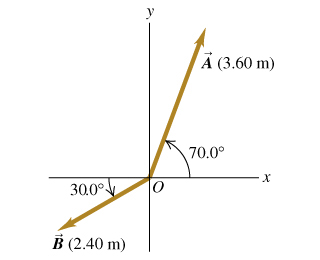# Problem: a) Write the vector  A  in the figure (Figure 1) in terms of the unit vectors  î  and  ĵ.b) Write the vector  B  in the figure in terms of the unit vectors  î  and  ĵ.c) Use unit vectors to express the vector  C , where  C  = 3.00A −4.00B  .d) Find the magnitude of  C .e) Find the direction of  C .

###### FREE Expert Solution

Vector components:

Magnitude:

$\overline{)\mathbf{|}\stackrel{\mathbf{⇀}}{\mathbit{A}}\mathbf{|}{\mathbf{=}}\sqrt{{{\mathbit{A}}_{\mathbit{x}}}^{\mathbf{2}}\mathbf{+}{{\mathbit{A}}_{\mathbit{y}}}^{\mathbf{2}}}}$

Direction:

$\overline{){\mathbf{tan}}{\mathbit{\theta }}{\mathbf{=}}\frac{{\mathbit{A}}_{\mathbit{y}}}{{\mathbit{A}}_{\mathbit{x}}}}$

a)

Ax = (3.60) cos 70° = 1.23m

100% (217 ratings)###### Problem Details

a) Write the vector  A  in the figure (Figure 1) in terms of the unit vectors    and  .

b) Write the vector  B  in the figure in terms of the unit vectors    and  .

c) Use unit vectors to express the vector  C , where  C  = 3.00A −4.00B  .

d) Find the magnitude of  C .

e) Find the direction of  C .